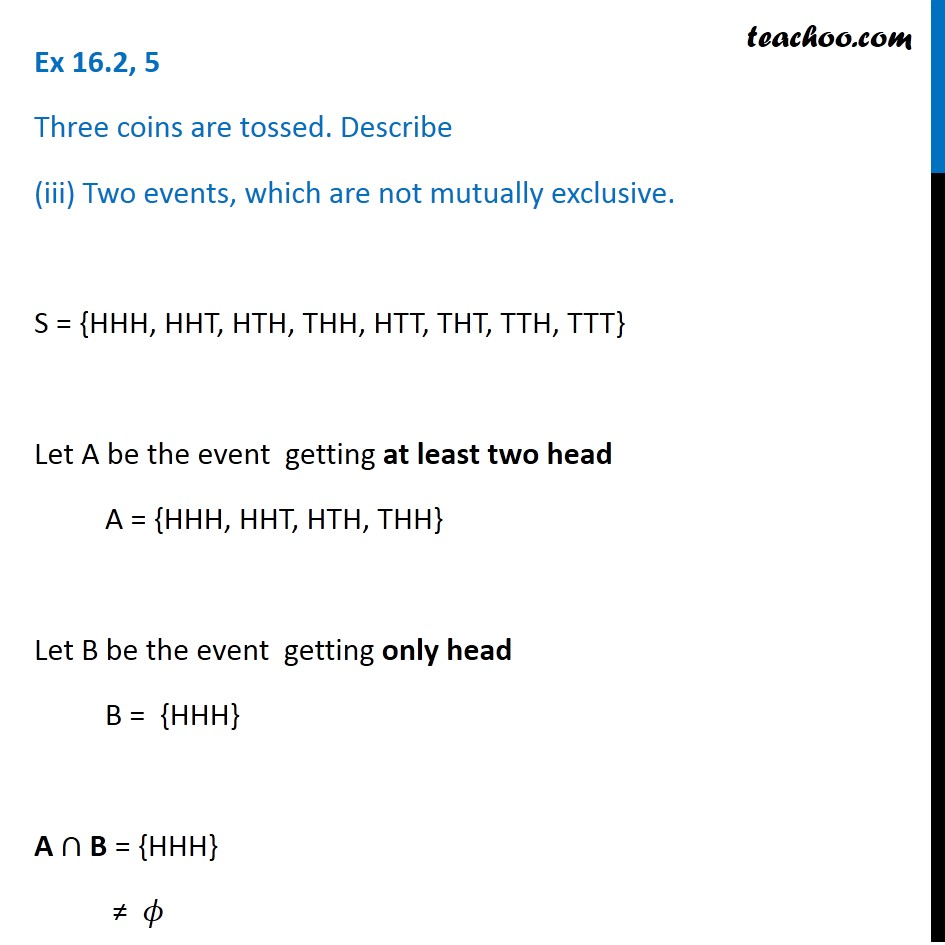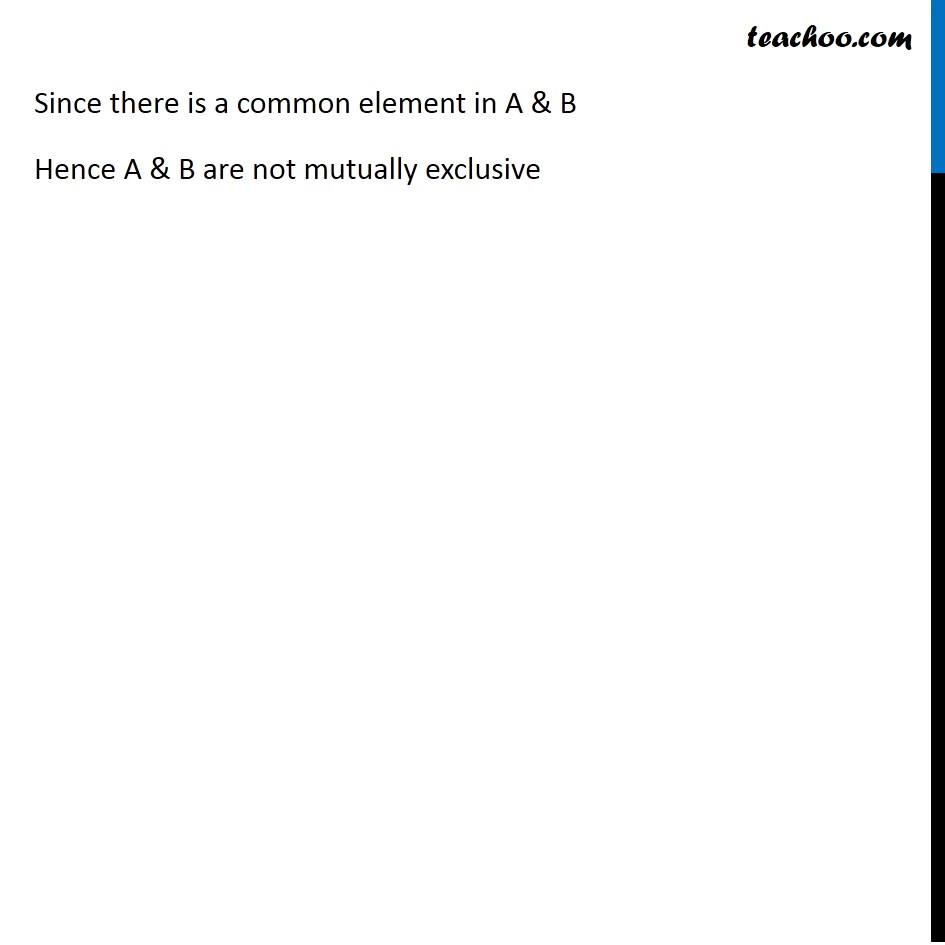1. Chapter 16 Class 11 Probability (Term 2)
2. Serial order wise
3. Ex 16.2

Transcript

Ex 16.2, 5 Three coins are tossed. Describe (iii) Two events, which are not mutually exclusive. S = {HHH, HHT, HTH, THH, HTT, THT, TTH, TTT} Let A be the event getting at least two head A = {HHH, HHT, HTH, THH} Let B be the event getting only head B = {HHH} A ∩ B = {HHH} ≠ 𝜙 Since there is a common element in A & B Hence A & B are not mutually exclusive